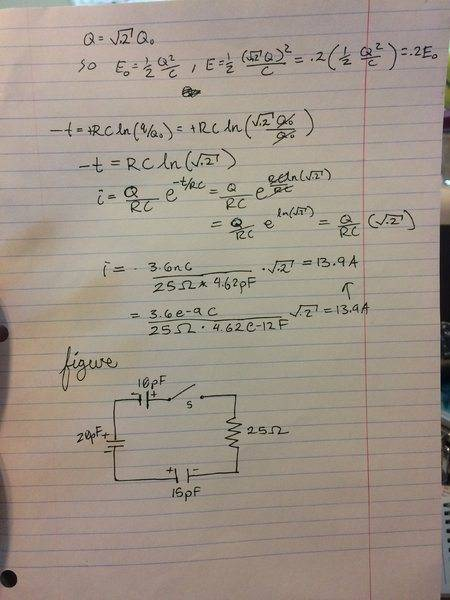# Need help finding current on RC circuit

• Ruskointhehizzy
In summary, the current in the circuit at the instant that the capacitors have lost 80.0% of their initial stored energy is 13.9A.f

## Homework Statement

In the circuit shown in the figure each capacitor
initially has a charge of
magnitude 3.60nC on its plates. After the switch S is closed, what will be the
current in the circuit at the instant that the capacitors
have lost 80.0% of their initial stored energy?
the shown 3 capacitors are 10, 15, and 20 pF and the one resistor has 25ohms - all in series with one switch S.

## Homework Equations

E = 1/2Q^2/c = QV/2
V_c(t)=Q/c * (1-e^(-t/RC))
i = Q/RC * e^(-t/RC)

I have since solved this but I would really appreciate it if I could get this checked for correctness.
Thank you.#### Attachments

Last edited:
I think I have figured out my mistake but please can I still get a check to see if my solution is correct?
first I find what Q needs to be to get .2E
to do this I say:
E0 = ½Q2/C, E = ½ ( √.2 Q )2/C = .2(½ Q2/C) = .2E0
I then use this to find -t
-t = RCln(Q/Q0) = RCln(√.2Q0/Q0) = RCln(√.2)
then finally
i = - Q/RC * e-t/RC = Q/RC * eRCln(√.2)/RC = Q/RC * eln√.2 = = -(3.6nC/(25Ω*4.62pF)) * √.2
i = = -3.6e-9C * √.2 / ( 25Ω * 4.62e-12F) = 13.9A

We can't see your images, which is probably why nobody has responded.

•Ruskointhehizzy
We can't see your images, which is probably why nobody has responded.
Yeah I tried to post a image with a link to my google drive but it didn't let me - then when I tried to remove it wouldn't let me.
I finally resorted to posting it in the comments lmao.
Did you have a chance to check over my solution? I hope I got this correct I've been stuck on it for awhile

I don't see the figure anywhere. Have you tried just dragging the figure into the comment entry window?

I don't see the figure anywhere. Have you tried just dragging the figure into the comment entry window?
oh that worked thank you

that was just my first wrong attempt - I will change the op